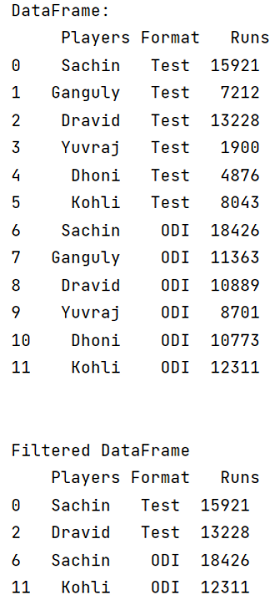# How to select with complex criteria from pandas DataFrame?

Given a DataFrame, we have to select with complex criteria from it. By Pranit Sharma Last updated : September 20, 2023

Pandas is a special tool that allows us to perform complex manipulations of data effectively and efficiently. Inside pandas, we mostly deal with a dataset in the form of DataFrame. DataFrames are 2-dimensional data structures in pandas. DataFrames consist of rows, columns, and the data. DataFrame can be created with the help of python dictionaries or lists but in the real world, CSV files are imported and then converted into DataFrames. Sometimes, DataFrames are first written into CSV files.

## Problem statement

Given a DataFrame, we have to select with complex criteria from it.

## Selecting with complex criteria from pandas DataFrame

For this purpose, we will use the DataFrame.query() method inside the DataFrame.loc[] property by passing the condition along with the .index() method. The DataFrame.query() method allows us to apply any condition or query in the DataFrame. It returns the data after filtering it through the query. Consider the below syntax of the method:

```DataFrame.query(expr, inplace=False, **kwargs)
```
Note

To work with pandas, we need to import pandas package first, below is the syntax:

```import pandas as pd
```

Let us understand with the help of an example.

## Python program to select with complex criteria from pandas DataFrame

```# Importing pandas package
import pandas as pd

# creating a dictionary of student marks
d = {
"Players":['Sachin','Ganguly','Dravid','Yuvraj','Dhoni','Kohli',
'Sachin','Ganguly','Dravid','Yuvraj','Dhoni','Kohli'],
"Format":['Test','Test','Test','Test','Test','Test',
'ODI','ODI','ODI','ODI','ODI','ODI'],
"Runs":[15921,7212,13228,1900,4876,8043,
18426,11363,10889,8701,10773,12311]
}

# Now we will create DataFrame
df=pd.DataFrame(d)

# Viewing the DataFrame
print("DataFrame:\n",df,"\n\n")

# Applying a query
df1 = df.loc[df.query(
'Runs >= 12000'
).index]

# Display df1
print("Filtered DataFrame\n",df1)
```

### Output

The output of the above program is: